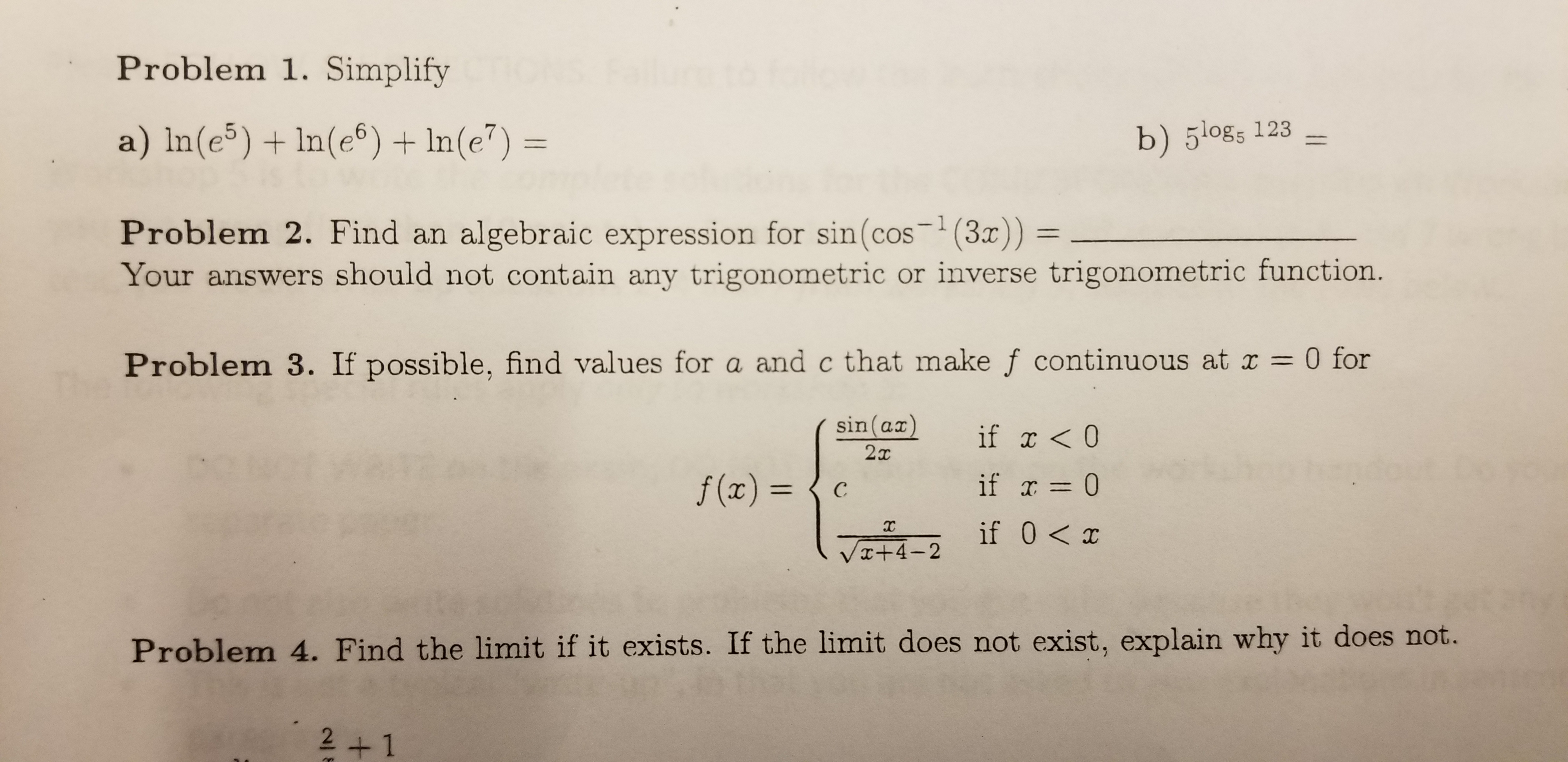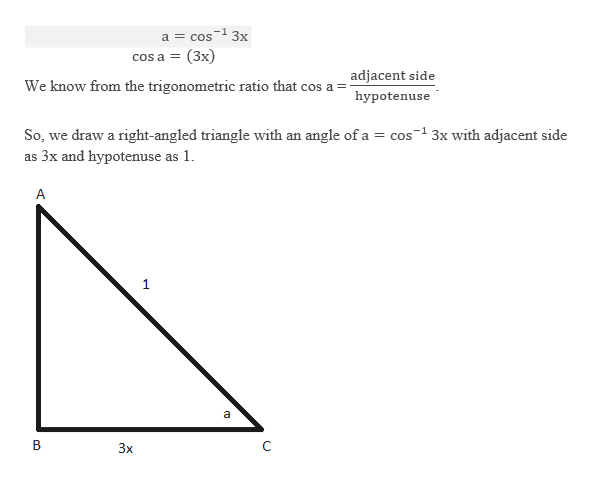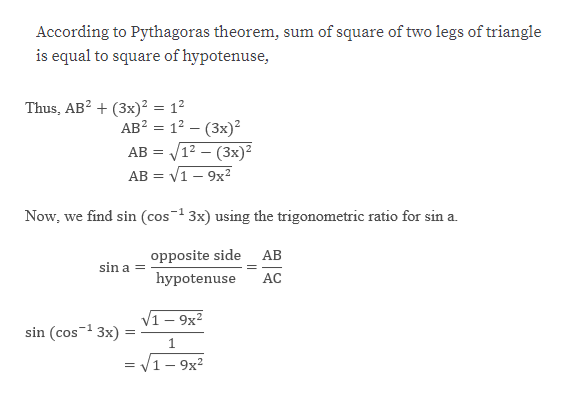# Problem 1. Simplifya) In(e5) In(e6) In(e7) =b) 5l0gs 123algebraic expression for sin(cos (3)) =Your answers should not contain any trigonometric or inverse trigonometric function.- 1Problem 2. Find an0 forProblem 3. If possible, find values for a and c that make f continuous at r1sin (ax)if x

Question
22 viewshelp_outlineImage TranscriptioncloseProblem 1. Simplify a) In(e5) In(e6) In(e7) = b) 5l0gs 123 algebraic expression for sin(cos (3)) = Your answers should not contain any trigonometric or inverse trigonometric function. - 1 Problem 2. Find an 0 for Problem 3. If possible, find values for a and c that make f continuous at r 1 sin (ax) if x<0 2т if 0 f(x) = с if 0 x VI+4-2 Problem 4. Find the limit if it exists. If the limit does not exist, explain why it does not. 2+1 fullscreen
check_circle

Step 1

To find:

Step 2

Let us assume the following,help_outlineImage Transcriptionclose1 3x 1 cos a (3x) COs a = adjacent side We know from the trigonometric ratio that cos a hypotenuse So, we draw a right-angled triangle with an angle of a cos3x with adjacent side as 3x and hypotenuse as 1 A 1 a В C Зх fullscreen
Step 3

Note:

...help_outlineImage TranscriptioncloseAccording to Pythagoras theorem, sum of square of two legs of triangle is equal to square of hypotenuse, Thus, AB2(3x)2 = 12 AB2 12 (3x) 12 - (3x)2 V1-9x2 AB = АВ Now, we find sin (cos1 3x) using the trigonometric ratio for sin a. АВ opposite side sin a = hypotenuse АC 1-9x2 sin (cos 3x) -1 1 = V1 -9x2 fullscreen

### Want to see the full answer?

See Solution

#### Want to see this answer and more?

Solutions are written by subject experts who are available 24/7. Questions are typically answered within 1 hour.*

See Solution
*Response times may vary by subject and question.
Tagged in
MathCalculus

### Other